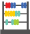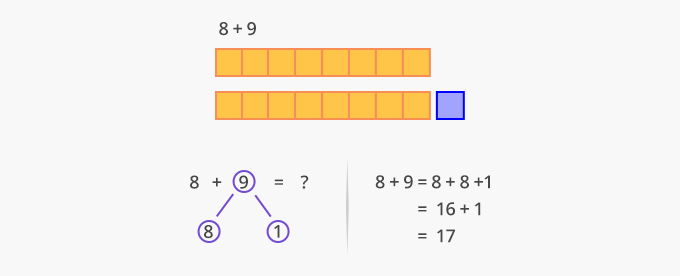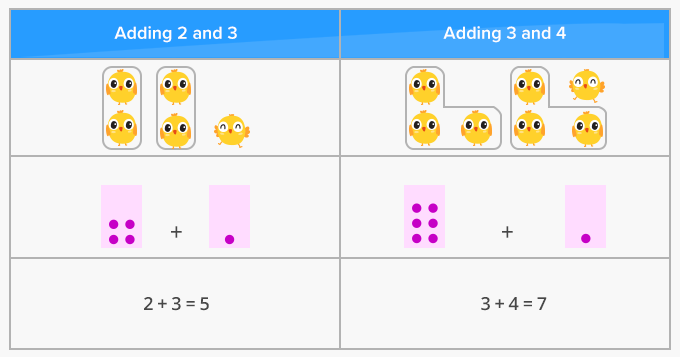# Doubles Plus 1 - Definition with Examples

The Complete K-5 Math Learning Program Built for Your Child

• 30 Million Kids

Loved by kids and parent worldwide

• 50,000 Schools

Trusted by teachers across schools

• Comprehensive Curriculum

Aligned to Common Core

##Let's learn!

What is doubles plus 1?
Doubles plus 1 is a strategy used to add two consecutive numbers that is, when they are next to each other.
We simply add the smaller number twice or double it and then,  add 1 to it, to get the final result.

Here, for example, consecutive numbers 8 and 9 have been added using the doubles plus one strategy.Here are more examples of the double plus 1 strategy.Fun Facts Doubles plus 1 and doubles minus 1 are also called near doubles strategies.

##Let's sing!

Adding five and six; there’s no trouble!
Just make the smaller number double,
Then add 1 to the double of the bubbles.

##Let's do it!

Have fun with your child by asking them to find the doubles of numbers you say and then use double plus 1 and doubles minus 1 strategies to find the sum of consecutive numbers between 1 and 20.

##Related math vocabulary

Won Numerous Awards & Honors